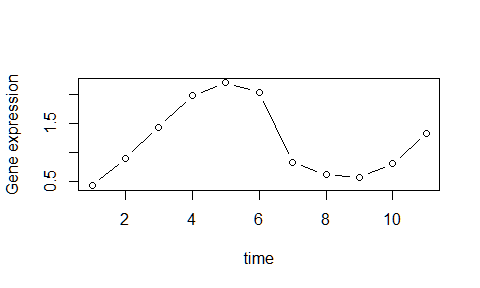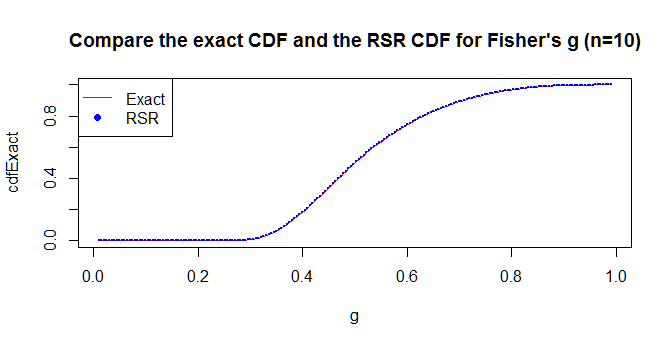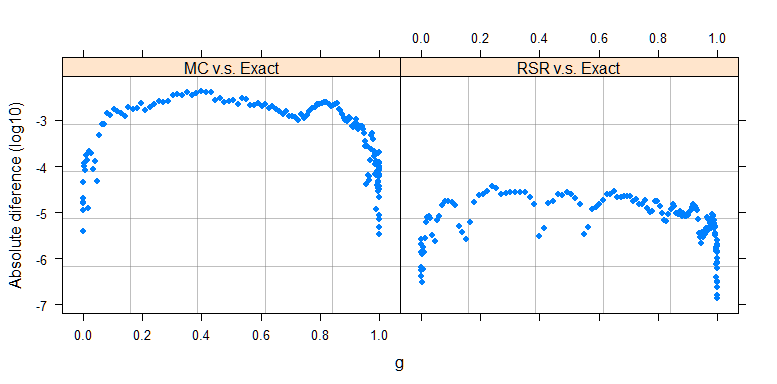Introduction to the ptest package

Abstract

This vignette introduces the functionality of the ptest package for fitting harmonic models and detecting the periodicty for short time series.

Motivation

Currently there are many statistical tests available for detecting the periodicity in microarray gene expression data. However, methods like the Fisher’s g test proposed by (Wichert, Fokianos, and Strimmer 2004) might fail to detect the periodicity as it only consider the Fourier frequencies, and methods like the robust g test (Ahdesmäki et al. 2005) lacks of the computational efficiency as the test requires time-consuming Monte Carlo simulations.

The figure below shows the gene expression measured at 11 hourly intervals for gene ORF06806, which is apparently periodic with period of about 8.4 hours. About 7 other genes (out of 3000 available) show strong periodicity that is not detected by Fisher’s g or other tests in current use for detecting periodicity in time series microarrays (Wichert, Fokianos, and Strimmer 2004).

#> Periodicity tests in short time seriesGene ORF06806

We introduce ptest as an efficient and elementary tool for modeling and testing periodicity in short time series, which aims to deal with the above obstacles. It includes serveral periodicity tests, whose p-value can be obtained instantly and accurately by the response surface regression (RSR) method (MacKinnon 2000). It also includes a likelihood ratio test based on the harmonic regression model with the estimated frequency searched among $$\mathcal{E}_{n}=\{ j/101\mid j=1,\dots,50\wedge j/101\ge1/n\}$$, which allows for detecting both Fourier frequencies and the non-Fourier frequencies.

Harmonic model

For the introduced methods, we assume there are no missing values in the data and the model for the periodic time series is, $z_{t}=\mu+A\cos(2\pi\lambda t)+B\sin(2\pi\lambda t)+\varepsilon_{t},\ t=1,...,n,$

where $$\epsilon_{t}$$ is an i.i.d. noise sequence with mean 0 and variance $$\sigma^{2}$$, the frequency $$\lambda$$ of the sinusoidal wave is restricted to the range $$0\le\lambda\le0.5$$ to avoid the aliasing (indeterminacy) the sinusoidal function, $$A$$ and $$B$$ are some constants.

Our objective is to perform a hypothesis test for detecting the periodicity, $\text{H}_{0}:\lambda=0\ \text{versus}\ \text{H}_{1}:\lambda>0.$

Fitting harmonic models with ptest

The function fitHReg() provides maximum likelihood estimations (MLE) for fitting the harmonic model. The default maximization of the likelihood is performed by the enumerative algorithm but an exact non-linear least square option is also provided. Here is a brief example to do the estimations (See below).

#simulate a harmonic series
set.seed(193)
#Non-Fourier frequency
z <- simHReg(n = 14, f=2/10, A = 2, B = 1, model="Gaussian",sig=1)
fitHReg(z,algorithm="enumerative")
#> $coefficients #> mu A B #> 0.2930025 2.2207896 0.5829751 #> #>$residuals
#>            1            2            3            4            5
#>  0.086619003 -0.432458497  0.901698244 -0.740022388  0.002371533
#>            6            7            8            9           10
#> -1.416528621 -0.199844613  0.401282041  0.136717738  0.343483073
#>           11           12           13           14
#>  1.194367583  0.542615248 -0.884524542  0.064224200
#>
#> $Rsq #>  0.8428944 #> #>$fstatistic
#>    value    numdf    dendf
#> 29.50829  2.00000 11.00000
#>
#> $sigma #>  0.7631791 #> #>$freq
#>  0.1980198
#>
#> $LRStat #>  25.91172 #> #> attr(,"class") #>  "HReg" fitHReg(z,algorithm = "exact") #>$coefficients
#>        mu         A         B
#> 0.3292958 2.3115821 0.1579380
#>
#> $residuals #> 1 2 3 4 5 6 #> 0.3744325 -0.2111299 0.7795052 -0.9979541 -0.1007008 -1.3723056 #> 7 8 9 10 11 12 #> -0.1745866 0.3832477 0.1481103 0.3270874 1.0308152 0.3339204 #> 13 14 #> -0.8603981 0.3399565 #> #>$Rsq
#>  0.8524324
#>
#> $fstatistic #> value numdf dendf #> 31.77105 2.00000 11.00000 #> #>$sigma
#>  0.7396498
#>
#> $freq #> lambda #> 0.1943304 #> #>$LRStat
#>  26.78856
#>
#> attr(,"class")
#>  "HReg"

The recommended package boot may be used with fitHReg() to perform a bootstrap significance test for periodicity.

#Bootstrap the series under the null hypothesis: No periodicity
set.seed(193)
library(boot)
LRStat <- function(d, i){
fitHReg(d[i],algorithm = "enumerative")$LRStat } bootResult <- boot(data = z, statistic = LRStat, R = 1000) bootResult #> #> ORDINARY NONPARAMETRIC BOOTSTRAP #> #> #> Call: #> boot(data = z, statistic = LRStat, R = 1000) #> #> #> Bootstrap Statistics : #> original bias std. error #> t1* 25.91172 -16.83044 3.292104 #Compute the p-value mean(bootResult$t>=fitHReg(z,algorithm="enumerative")$LRStat) #0.001 #>  0.001 Methods for detecting periodicity In order to detect whether the series is periodic or not, ptest provides two kinds of tests using the function ptestReg() and the function ptestg(). ptestReg() use likelihood ratio tests under the Gaussian or the Laplace assumptions, while ptestg() provides three methods based on the periodograms. Both methods are implemented with the RSR method implemented for efficiently obtaining accurate p-values. Likelihood ratio tests using ptestReg() ptestReg() performs two kinds of likelihood ratio tests based on two different assumptions (Gaussian or Laplace) and the p-value computation is efficiently improved by the RSR method. Hence the p-value of the test can be instantly obtained without doing a Monte Carlo simulation and the precision is better than doing limited time of Monte Carlo simualtions in a personal computer. Here is a brief example. ptestReg(z,method = "LS") #Normal likelihood ratio test #>$obsStat
#>  25.91172
#>
#> $pvalue #> 1 #> 0.00107849 #> #>$freq
#>  0.1980198
#>
#> attr(,"class")
#>  "Htest"
ptestReg(z,method = "L1") #Laplace likelihood ratio test
#> $obsStat #>  13.91677 #> #>$pvalue
#>           1
#> 0.002480358
#>
#> $freq #>  0.1980198 #> #> attr(,"class") #>  "Htest" Periodogram-based tests using ptestg The g statistic was initially proposed by (Fisher 1929) and is used to test the periodicity in microarray data in (Wichert, Fokianos, and Strimmer 2004). It is defined as a ratio of the maximum periodogram to the summation of all periodograms evaluated at the Fourier frequencies, \begin{equation} g=\frac{\max_{j}I(\lambda_{j})}{\sum_{j=1}^{m}I(\lambda_{j})},\label{eq:g-statistic} \end{equation} where $$m$$ is $$(n-1)/2$$ or $$(n-2)/2$$ according to $$n$$ is odd or even and $$I(\lambda_{j})$$ is the periodogram evaluated at the Fourier frequencies $$\lambda_{j}=j/n$$, $$j=1,...,[n/2]$$ and $$[x]$$ denotes the integer part of x. ptestg(z,method="Fisher") #>$obsStat
#>  0.6388557
#>
#> $pvalue #>  0.03685997 #> #>$freq
#>  0.2142857
#>
#> attr(,"class")
#>  "Htest"

The other two periodogram-based tests are the modifications of the Fisher’s g test. For the robust g test prposed by (Ahdesmäki et al. 2005), it replaces periodogram spectral estimator with an alternative rank-based robust estimator. The original robust g test staitstic is evaluated by enlarging twice the searching region of the fourier frequencies, but we also made an alternative option that the evaluated frequencies are $$\cal{E}_{n}$$.

ptestg(z,method="robust")
#> $obsStat #>  0.3570414 #> #>$pvalue
#>          1
#> 0.03395255
#>
#> $freq #>  0.1785714 #> #> attr(,"class") #>  "Htest" #extend the frequency searching region to be En ptestg(z,method="extendedRobust") #>$obsStat
#>  0.1115661
#>
#> $pvalue #> 1 #> 0.04370089 #> #>$freq
#>  0.1980198
#>
#> attr(,"class")
#>  "Htest"

For the extended Fisher’g test statistic, it simply extends the Fisher’s g test by enlarging the searching region of the frequency from the fourier frequencies to $$\mathcal{E}_{n}$$.

ptestg(z,method="extended")
#> $obsStat #>  0.1107367 #> #>$pvalue
#>          1
#> 0.03785992
#>
#> $freq #>  0.1980198 #> #> attr(,"class") #>  "Htest" Motivating Examples Here we introduce some examples to stress the superiority of ptest. Efficiency with the response surface regression method To see the efficiency of ptest, we compare the computation time of the robust g test in the GeneCycle package and the the robust g test in ptest. Here is the result on a single local machine with two cores Intel(R) Core(TM) i7-4500U CPU @ 1.80 GHz 2.40 GHz, Windows 10 64-bit system, and R 3.3.1. Notice that here we only present the computation time of GeneCycle based on $$10^5$$ MC simulations, which is also the original set-up. But to achieve the same accuracy as the RSR method, it needs $$200\times 10^5$$ simulations, which is the simulation times used in the RSR method for the robust g in ptest. # Effiency Comparison for testing 10^5 series with length 20 set.seed(193) X <- matrix(rnorm(20*10^5),nrow = 10) ## MC method for the robust g test library(GeneCycle) # # The original robust g test performs 10^5 MC simualtions, # # but we need 100*10^5 to achieve the same accuracy as # # the RSR method. # # This process is time-consuming. # t1 <- proc.time() # RX <- robust.spectrum(X) # pval1 <- robust.g.test(RX) # t1 <- proc.time()-t1 # # t1 ##> user system elapsed ##> 343.42 2.09 348.78 #unlink("g_pop_length_10.txt") # delete the external files hence for achieving the same accuracy as the below robust g test with the RSR method, it takes $$348.78\times 200/3600 \ \text{hours} = 19.4\ \text{hours}$$. Moreover, the memory needed is 99kb and hence the memory needed for the whole simulation will be almost 1GB. Fortunately, with the help of the RSR methods, those time-consuming simulations can be skipped. ## RSR method for the robust g test library(ptest) t2 <- proc.time() pval2 <- ptestg(X,method="robust",multiple = TRUE) t2 <- proc.time()-t2 t2 # user system elapsed # 208.97 0.17 218.42 Accuracy with the response surface regression method To see the accuracy of computed p-values from the RSR method, the exact cumulative distribution function of the Fisher’s g is compared to its cumulative distribution function derived from the RSR method. For this experimental purpose, ptestg() provides an option for computing the p-value of the Fisher’g test with the RSR method. #For a series of length 10, it is by the definition of the g statistic that #the range of g should be between 1/n and 1. n <- 10 g <- seq(0.01,0.99,length.out = 200) #Compute the CDF from the exact distribution and the RSR method cdfExact <- pFisherg(g,n,method="exact") cdfRSR <- pFisherg(g,n,method="RSR") plot(g,cdfExact,col="red",type="l", cex=1.2, main = "Compare the exact CDF and the RSR CDF for Fisher's g (n=10)") lines(g,cdfRSR,col="blue",type="b",pch=19,cex=0.3) legend("topleft",legend = c("Exact","RSR"), col = c("red","blue"), lty = c(1,NA), pch = c(NA, 19))The absolute difference (in log 10 scale) between the exact distribution and the RSR/MC distribution is also shown. MC represents the emiprical distribution obtained from $$10^5$$ Monte Carlo simulations of the Fisher’s g statistics. Usually this simulation step takes a long time (i.e., the robust g statistic), but since the g statistic is computed efficiently by the Fast Fourier transfomations, doing $$10^5$$ simulations is still acceptible. The figures shows that the RSR distribution is more accurate than the Monte Carlo method. set.seed(193) zsim <- matrix(rnorm(10*10^5),nrow=10,ncol=10^5) gSample <- ptestg(zsim,method="Fisher",multiple = TRUE)$obsStat
distMC <- ecdf(gSample)
cdfMC <- distMC(g)

#It is not of interest to compare thoes values which are less than 0.00001
#or larger than 0.99999 as the 10^6 Monte Carlo simulations here does not
#enable a reliable estimation for them.
pos <- which( (cdfExact>=0.00001)&(cdfExact<=0.99999) )
diff1 <- log(abs(cdfExact[pos]-cdfRSR[pos]),base = 10)
diff2 <- log(abs(cdfExact[pos]-cdfMC[pos]),base = 10)
comFig <- data.frame(cdfExact = cdfExact[pos],
diff=c(diff1,diff2),
type=rep(c("RSR v.s. Exact","MC v.s. Exact"),
each=length(diff1)))

library(lattice)
#>
#> Attaching package: 'lattice'
#> The following object is masked from 'package:boot':
#>
#>     melanoma
ans <- xyplot(diff ~ cdfExact | type, data=comFig,
panel=function(x,y){
panel.grid(h=4, v= 4,col=rgb(0.5,0.5,0.5,0.5))
panel.xyplot(x, y, pch=16,cex=0.7)
}, ylab="Absolute diference (log10)", xlab="g")
ansAbsolute difference between CDFs

Might fail to detect non-fourier periodicities

Let’s come back to the example of gene ORF06806. It is apparently periodic with period of about 8.4 hours, approximately with a non-Fourier frequency $$1/8.4 = 0.1190$$. But the Fisher’s g failed to detect this periodicity.

data(Cc)
x <- Cc[which(rownames(Cc)=="ORF06806"),]
plot(1:length(x),x,type="b",
xlab="time",ylab="Gene expression")Gene ORF06806

ptestg(x,method="Fisher") #Fail to detect
#> $obsStat #>  0.6067813 #> #>$pvalue
#>  0.1195382
#>
#> $freq #>  0.09090909 #> #> attr(,"class") #>  "Htest" ptestg(x,method="robust") #>$obsStat
#>  0.4659265
#>
#> $pvalue #> 1 #> 0.008630823 #> #>$freq
#>  0.1363636
#>
#> attr(,"class")
#>  "Htest"
ptestg(x,method="extended")
#> $obsStat #>  0.1194861 #> #>$pvalue
#>          1
#> 0.02176852
#>
#> $freq #>  0.1287129 #> #> attr(,"class") #>  "Htest" ptestReg(x,method="LS") #>$obsStat
#>  31.42167
#>
#> $pvalue #> 1 #> 0.0003234099 #> #>$freq
#>  0.1188119
#>
#> attr(,"class")
#>  "Htest"
ptestReg(x,method="L1")
#> $obsStat #>  17.57679 #> #>$pvalue
#>            1
#> 0.0003295722
#>
#> $freq #>  0.1287129 #> #> attr(,"class") #>  "Htest" X <- Cc[complete.cases(Cc),] pvalue <- ptestg(t(X),method="Fisher",multiple = TRUE) head(sort(pvalue$pvalue))
#>  2.034930e-05 2.682115e-05 6.673249e-05 7.087520e-05 9.244960e-05
#>  9.574902e-05

Reference

Ahdesmäki, Miika, Harri Lähdesmäki, Ron Pearson, Heikki Huttunen, and Olli Yli-Harja. 2005. “Robust Detection of Periodic Time Series Measured from Biological Systems.” BMC Bioinformatics 6 (1). BioMed Central Ltd: 117.

Fisher, Ronald Aylmer. 1929. “Tests of Significance in Harmonic Analysis.” Proceedings of the Royal Society of London. Series A, Containing Papers of a Mathematical and Physical Character. JSTOR, 54–59.

MacKinnon, James G. 2000. “Computing Numerical Distribution Functions in Econometrics.” In High Performance Computing Systems and Applications, 455–71. Springer.

Wichert, Sofia, Konstantinos Fokianos, and Korbinian Strimmer. 2004. “Identifying Periodically Expressed Transcripts in Microarray Time Series Data.” Bioinformatics 20 (1): 5–20. doi:10.1093/bioinformatics/btg364.